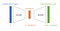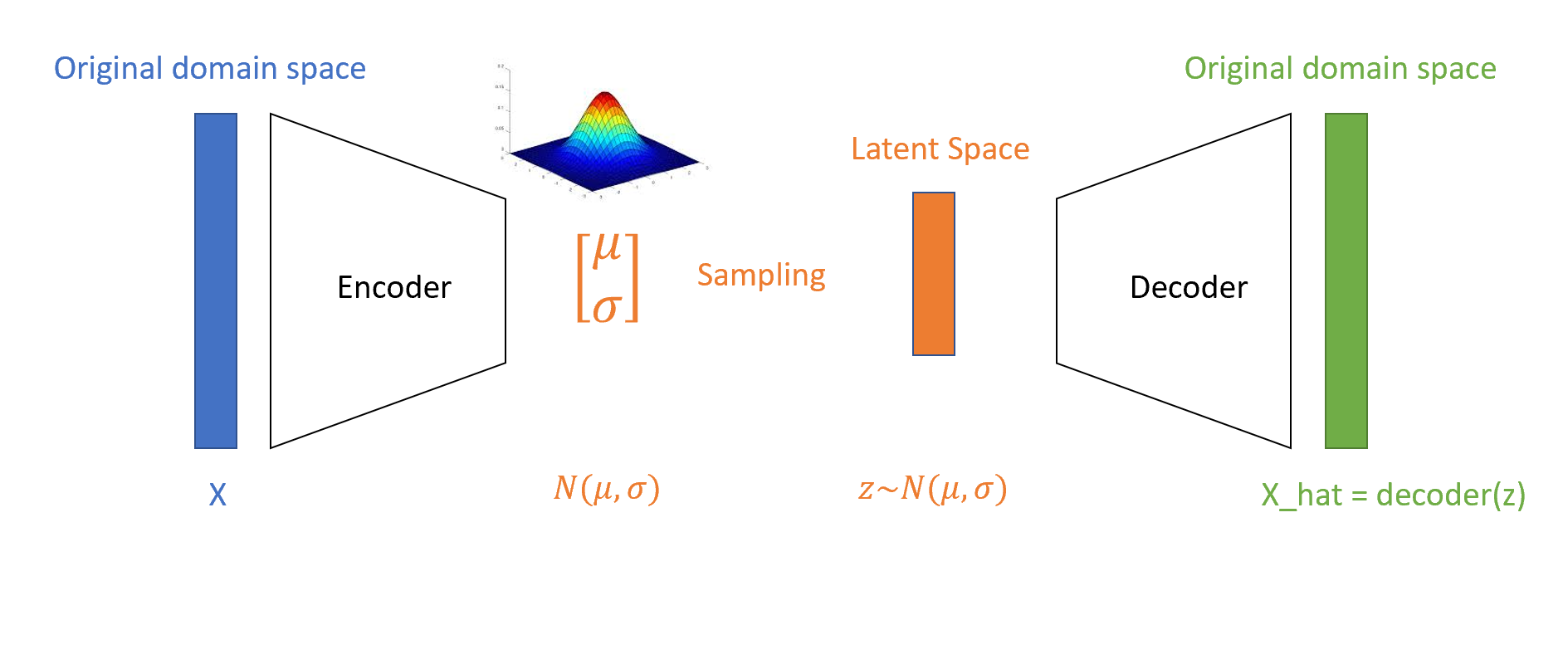# Variational Autoencoders in Computer Vision

In my previous article, I’ve been talking about Autoencoders and their applications, especially in the Computer Vision field.

Generally speaking, an autoencoder (AE) learns to represent some input information (in our case, images input) by compressing them into a latent space, then reconstructing the input from its compressed form to a new, auto-generated output image (again in the original domain space).

In this article, I’m going to focus on a particular class of Autoencoders which have been introduced a bit later, that is that of Variational Autoencoders (VAEs).

VAEs are a variation of AEs in the sense that their main job is that of learning a probabilistic model, living in the latent feature space of the compressed image, from which one can sample and generate new images.

Let’s have a look at the main differences between the two methods:

• AEs learn a compressed representation of images (of course, it holds also for other field, like NLP — in that case, input data will be texts). It does so by first compressing the image via an encoder network and then decompressing it with a decoder network back to the original domain space. The goal is having a decompressed image that is as close as possible to the original one.How Autoencoders work
• VAEs, on the other hand, learn the parameters (mean and variance vector) of a probability distribution (multivariate gaussian) representing data. Once we have this distribution parametrized, we can actually sample from it and generate new images. In that sense, VAEs are generative models, like GANs (you can read more about GANs here).

More specifically, the encoder component of VAEs maps each pixel into a mean and variance vector, which are the parameter of the Multivariate Gaussian. What about the covariances? Well, VAEs assume no correlation among dimensions in the latent space, hence the covariance matrix is diagonal — we only have to think about mean and variance.

Let’s have a look at all the steps (more details here):

• we first initialize an encoder network that maps each pixel into a mean and variance vector: z_mean and z_log_sigma.
• then, we sample random points z from the gaussian distribution with parameters z_mean and z_log_sigma, via z = z_mean + expo(z_log_sigma)*epsilon, where epsilon is a tensor sample from the standard normal distribution
• finally, a decoder network maps these latent space points back to the original domain space.How a VAE works

Another important difference between AEs and VAEs is the way their performance are measured. In the case of AEs, as mentioned above, the goal is that of having the deocded image as close as possible to the original one. As such, we want to minimize an objective function which compute the distance between the two images (namely, the RMSE, MSE…).

On the other hand, when we train a VAE, we need to consider two error components:

• reconstruction error. It refers to the similarity between output and input images (like in AEs). Also in this case, a distance loss function like MSE can be employed;
• regularization error. It refers to the organization of the latent space of the probability distribution. The idea is that of forcing the anew parametrized distribution to behave and look like a standard normal. To do so, we use a metric which computes how two probability distributions differ among each others (in this case, the two distributions are the one derived from the encoder and a standard normal). This metric is called Kullback–Leibler divergence. The reason why we want to regularize our latent space is because we want it to fulfill the two properties of continuity and completeness.

The final error is nothing but a weigthed sum of the two (weights’ choice is upon the scientist).

Since the introduction of GANs, the new state of the art, VAEs have been contributing less to the generative techniques in computer vision. Nevertheless, they are still precious in many use cases. Namely, as they model a probabilistic distribution, it can be a great insight into input images, namely to look for anomalies. Or even more, you can build a customized network which combines both GANs and VAEs.

Cloud Specialist at @Microsoft | MSc in Data Science | Machine Learning, Statistics and Running enthusiast

## More from Valentina Alto

Cloud Specialist at @Microsoft | MSc in Data Science | Machine Learning, Statistics and Running enthusiast